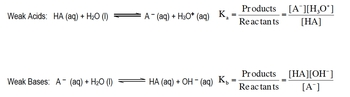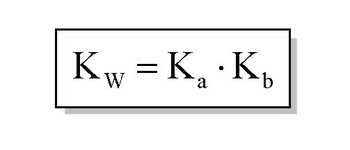Start typing, then use the up and down arrows to select an option from the list.# GOB Chemistry

Learn the toughest concepts covered in your GOB - General, Organic, and Biological Chemistry class with step-by-step video tutorials and practice problems.

Acids and Bases

Associated with any weak acid or weak base is a Ka or Kb value respectively.

## Acid and Base Dissociation Constants.

1

#### concept

Ka and Kb Concept 11m
Play a video:
Hey, guys, In this new video, we're gonna take a look at the acid and base Constance. So as you guys can know from looking at the rules for identifying compounds as either acids or bases, you should realize that there's a lot more weak species out there than there are strong ones we need to realize is thes week. Species have constants associated with them, so we're gonna say here, consider a week mono product H a acid mono product just means it has one h positive. And here we're looking at how it ionized is in water. So basically, H is the acid, so it's gonna give away in H positive to the water. The water accepts the h positive. So that's how it becomes H 30 plus the acid loses an h positive, and that's how it becomes a minus. Now what we're gonna say here the equilibrium expression equals products overreact. It's remember, we've talked about the equilibrium expression before it looks at all phases of matter, except for liquids and solids, so liquids and solids will not be included. And here we have a liquid. So we're gonna ignore it when we do the equilibrium expression. So it's simply gonna become a minus times H 30 plus over H A

Weak acids possess a Ka value less than 1, while weak bases possess a Kb value less than 1. The equilibrium expressions of Ka and Kb are the same as other equilibrium constants we’ve seen.2

#### concept

Ka and Kb Concept 23m
Play a video:
Now we're gonna say that K represents our acid association constant. And it basically measures how strong or weak acids are. And we're gonna say weak acids use K a week basis. Don't use K A Instead, they use K B. So this is our based association constant. What we're gonna say here is their relationship to each other. K and K B can be expressed by this formula. K w equals K a times K B. And as you realize this K w is the same k w we saw earlier in the earlier times, we saw it as K w equals h Positive times O H minus. It's the same exact kw. And we're going to say in general, the higher the K value than the stronger the acid, the stronger the acid, the greater the concentration of H plus. Now we're gonna say related to K is PK. And remember we talked about what p means. P means negative log. So PKK means negative log of K here. We're gonna say there's an inverse relationship. It's actually the lower the PK, the stronger the acid and the stronger the acid, the greater the H plus concentration now we're gonna say here is when it comes to a weak acid, we're gonna save weak ass. It tends to have k value less than one and a peek a value greater than one. Just realized we set inverse relationship. So okay, and pkr opposites. If one is up, the other one is down strong acids. Though strong acids tend tohave, Pekka is much greater than one. I mean, not PKs with K is much greater than one, and they tend to have PKK's lower than one PK that are negative. And remember, we should be able to establish a relationship between K m p k A. In the same way, we've been able to establish a relationship between H positive and pH because here we could just simply say K equals 10 to the negative p k. We could also say the same thing for PKB. P K B equals negative log of K B and therefore K B equals 10 to the negative PK. Just remember these relationships and how they affect the acidity of our compound. As long as you can remember these few quick, easy calculations, you'll be able to go from one to the other and one last thing. We could say here that the relationship from Peak to Peak A and P K B can be established by looking at this equation here by taking them negative log of K W K a n k b. We say that the equation becomes 14 equals P. K plus PKB Now that we've seen all these types of new variables and new types of equations attempt to do the practice question on the bottom here, I say If the KB of NH three is 1.76 times 10 to the negative five, what is the acid dissociation? Constant off the conjugate acid conjugate acid is a term we learned earlier. A few videos back, but here we were giving KB or has to find the acid association constant. So it's a simple conversion from one unit to another. So once you're done with that, click back on the explanation button and see how I approach this problem. Good luck, guys.

The greater the Ka value then the stronger the acid, while the greater the Kb the stronger the base. Ka and Kb are connected by the following equation:3

#### example

Ka and Kb Example 11m
Play a video:
Hey, guys, In this new video, we're gonna put to practice some of the concepts you learned about K and K B. So the first example says, knowing that HF has a larger or higher K value than ch three C O h, which is acetic acid. Determine if possible, in which direction the following equilibrium lies. All right. So we learned earlier that the higher your K value, the stronger you are as an acid. So HF has, ah higher K value. So it's a stronger acid than acetic acid is. So we're gonna say H f is the stronger acid. And again it's stronger because it has a higher K value and it's the stronger acid, so the acetic acid on the product side would be the weaker acid. Now we have to say on which side is the equilibrium lie. All you have to realize here is weaker means more stable in chemistry. Weaker just means less energy. Less energy means you're more stable, and the equilibrium will always lie on the side with the weaker acid. Because the weaker acid is the more stable acid, so are weaker. Acid is on the right side here, so we're gonna say that equilibrium lies to the right. It's a simple is that first you have to know that higher K means stronger acid and then just figure out which side the weaker acid would be on. Wherever the weaker ass it is, that's where your equilibrium will lie.
4
Problem

If the Kb of NH3 is 1.76 x 10-5, determine the acid dissociation constant of its conjugate acid.

5
Problem

Which Bronsted-Lowry base has the greatest concentration of hydroxide ions?

6
Problem

Which Bronsted-Lowry acid has the weakest conjugate base?